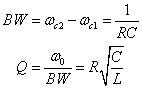##### Circuit Analysis For Dummies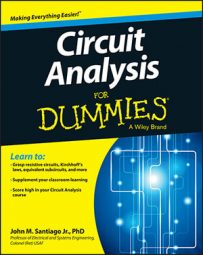There are many applications for an RLC circuit, including band-pass filters, band-reject filters, and low-/high-pass filters. You can use series and parallel RLC circuits to create band-pass and band-reject filters. An RLC circuit has a resistor, inductor, and capacitor connected in series or in parallel.

You can get a transfer function for a band-pass filter with a parallel RLC circuit, like the one shown here.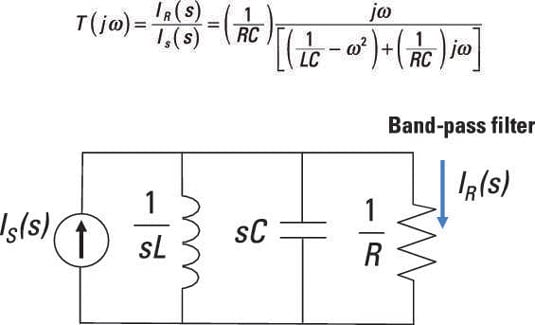You can use current division to find the current transfer function of the parallel RLC circuit. By measuring the current through the resistor IR(s), you form a band-pass filter. Start with the current divider equation: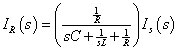A little algebraic manipulation gives you a current transfer function, T(s) = IR(s)/IS(s), for the band-pass filter: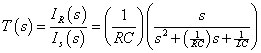Plug in s = jω to get the frequency response T(jω):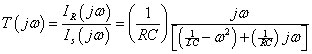This equation has the same form as the RLC series equations. For the rest of this problem, you follow the same process as for the RLC series circuit.

The transfer function is at a maximum when the denominator is minimized, which occurs when the real part of the denominator is set to 0. The cutoff frequencies are found when their gains |T(jωC)| = 0.707|T(jω)| or the –3 dB point. Therefore, ω0 is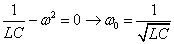The center frequency, the cutoff frequencies, and the bandwidth have equations identical to the ones for the RLC series band-pass filter.

Your cutoff frequencies are ωC1 and ωC2: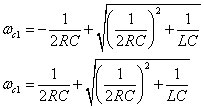The bandwidth BW and quality factor Q are